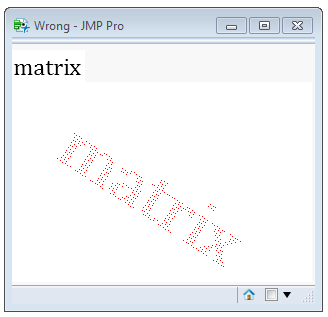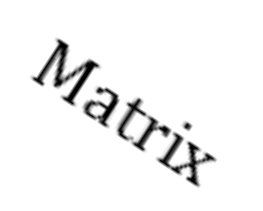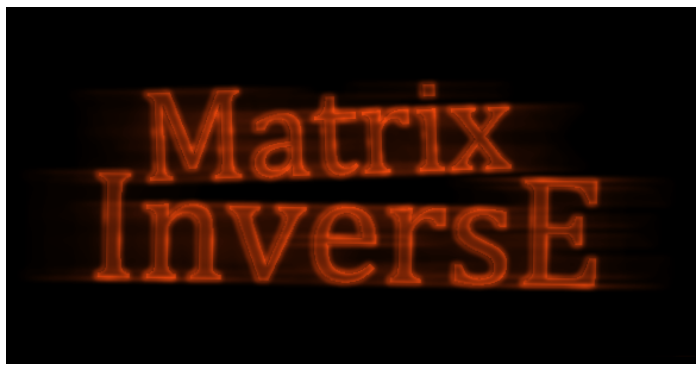Choose Language Hide Translation Bar
Rotation Matrix

The first time I tried to rotate and scale a bitmap, I did the obvious choice: take each pixel from the source matrix, transform it with a rotation and scale, and write the value to the transformed location in the destination.  The result looks like this:

``````bitmap = J( bmy = 200, bmx = 300, RGB Color( 1, 1, 1 ) );//white
scale = 3;
tranmat = (1 || 0 || 80) |/ (0 || 1 || 10) |/ (0 || 0 || 1); // 2D translation matrix
scalmat = (scale || 0 || 0) |/ (0 || scale || 0) |/ (0 || 0 || 1); // 2D scale matrix
angle = 33 * Pi() / 180;
sangle = Sin( angle );
cangle = Cos( angle );
rotamat = (cangle || -sangle || 0) |/ (sangle || cangle || 0) |/ (0 || 0 || 1); // 2D rotation matrix
forward = (tranmat * scalmat * rotamat)`; // transpose here
// make a sample image
testimage = Text Box( "matrix", <<setwrap( 1000 ), <<setfontsize( 18 ), <<Set Font Name( "Cambria" ) );
source = ((testimage << getpicture) << getpixels( "r" )); // red channel is enough
For( x = 1, x <= N Cols( source ), x += 1,
For( y = 1, y <= N Rows( source ), y += 1,
dest = (x || y || 1) * forward; // apply the forward transform matrix to each coordinate
If( source[y, x] != 1,
bitmap[dest, dest] = RGB Color( 1, 0, 0 )
);
)
);
oldimg = New Image( "rgb", {source, source, source} );
oldimg << scale( 1 );
newimg = New Image( bitmap );
newimg << scale( 1 );
New Window( "Wrong", oldimg, newimg );``````Transformed text has holes

Looks terrible. The correct way is to use the inverse of the transform matrix and ask "For each pixel in the destination bitmap, what pixel should I use from the source?" But the answer will almost always fall between four pixels. A reasonable answer is to use a weighted average of the four pixels, and more complicated answers might look like bi-cubic interpolation. This code uses a weighted average:

``````// this bitmap is used to build the image. drawtext will write
// each string into this bitmap.
bitmap = J( bmy = 300, bmx = 580, RGB Color( 1, 1, 1 ) );//white

// drawtext is a slow routine that draws a text string into a buffer,
// then samples that buffer to create a rotated, scaled copy in the
// bitmap buffer.
drawtext = Function(
{x = 0, y = 0, txt = "hello", degAngle = 0, xjust = 0, yjust = 1, fontsize = 99, scale = .5, textcolor = RGB Color( 0, 0, 0 )}, // parms
{// locals, initialized. Creates a transform matrix and its inverse
t = Text Box( txt, <<setwrap( 1000 ), <<setfontsize( fontsize ), <<Set Font Name( "Cambria" ) ) // "Rockwell" "Courier New" "Comic Sans MS" "Times New Roman"
, img = t << getpicture// gray scale antialiasing on edges, 1=white, 0=black, .4,.8,etc on transition
, mat = (img << getpixels( "r" )) // red channel is enough
, xsize = N Cols( mat ) // ~200 for width
, ysize = N Rows( mat ) // ~50 for height
// the forward matrix, that projects forward from img to bitmap, is built from pieces...
, justmat = (1 || 0 || (0 - xjust) * xsize) |/ (0 || 1 || (yjust - 1) * ysize) |/ (0 || 0 || 1) // xjust,yjust are 0...1 within text
, tranmat = (1 || 0 || x) |/ (0 || 1 || y) |/ (0 || 0 || 1) // 2D translation matrix
, scalmat = (scale || 0 || 0) |/ (0 || scale || 0) |/ (0 || 0 || 1) // 2D scale matrix
, angle = Pi() * degangle / 180, sangle = Sin( angle ), cangle = Cos( angle ), rotamat = (cangle || -sangle || 0) |/ (sangle || cangle || 0)
|/ (0 || 0 || 1) // 2D rotation matrix
// order is important. transpose is simpler than rearranging the matrices above.
, forward = (tranmat * scalmat * rotamat * justmat)` // transpose here
, reverse = Inverse( forward ) // <<< here's the inverse matrix
, sourcerect = // 2D coords of 4 corners of source image in "mat"
(0 || 0 || 1) |/ // upper left
(0 || ysize || 1) |/ // lower left
(xsize || ysize || 1) |/ // lower right
(xsize || 0 || 1)    // upper right
, destrect = sourcerect * forward// transformed 2D coords in destination
, destminx = Max( 1, Min( bmx, Floor( Min( destrect[0, 1] ) ) ) ) // make a rectangle
, destmaxx = Max( 1, Min( bmx, Ceiling( Max( destrect[0, 1] ) ) ) ) // holding the
, destminy = Max( 1, Min( bmy, Floor( Min( destrect[0, 2] ) ) ) ) // rotated destination
, destmaxy = Max( 1, Min( bmy, Ceiling( Max( destrect[0, 2] ) ) ) ) // rectangle, but clipped
, ri, gi, bi, ro, go, bo// colors
}, //
{ri, gi, bi} = Color To RGB( textcolor );
// use the inverse matrix on each destination pixel location to find a source pixel
// use the clipped destination rect. If the rotation angle is used, there will be points
// that fall outside the destination and points that fall outside the source. Clipping
// takes care of the points beyond the destination edge.
For( destx = destminx, destx <= destmaxx, destx += 1,
For( desty = destminy, desty <= destmaxy, desty += 1,
dest = destx || desty || 1; // 2D point in bitmap[]
source = dest * reverse;// typically not integer source coords
sourcelo = Floor( source ); // prepare for a linear interpolation between 4 source pixels
ratio = source - sourcelo; // x and y
If( 1 <= sourcelo < ysize & 1 <= sourcelo < xsize, // second clipping in the source bitmap
// q1 is the ratio of first row pixels, both columns. Ratio is fractional distance between columns.
q1 = mat[sourcelo, sourcelo] * (1 - ratio) + mat[sourcelo, sourcelo + 1] * (ratio);
// q2 is second row
q2 = mat[sourcelo + 1, sourcelo] * (1 - ratio) + mat[sourcelo + 1, sourcelo + 1] * (ratio);
// ratio is fractional distance between rows; combine the two ratios into q3
q3 = q1 * (1 - ratio) + q2 * (ratio);
mq3 = 1 - q3; // q3 and 1-q3 are used to blend new color with existing color...
{ro, go, bo} = Color To RGB( bitmap[desty, destx] ); // "old" existing color
// write the composited value back to the same pixel. ri,gi,gb are the constant
// text color, which is modified by q3, the blend amount
bitmap[desty, destx] = RGB Color( mq3 * ri + q3 * ro, mq3 * gi + q3 * go, mq3 * bi + q3 * bo );
);
)
);
);// drawtext

If( 1, // demo code
bitmap[0, 0] = RGB Color( 1, 1, 1 );
drawtext( 110, 40, "Matrix", 33, 0, 1, 18, 3 );
New Window( "Better", New Image( bitmap ) );
);

For( ipic = 1, ipic <= 2, ipic++, // do this twice, make two bitmaps, one without the "x"
bitmap[0, 0] = RGB Color( 1, 1, 1 ); // reset the bitmap to white at the start of each picture
// these two drawtext calls are carefully positioned and sized.
drawtext( 110, 40, If( ipic == 1, "Matrix", "Matri " ), -2/*tilt up*/, 0, 1, 99, 0.84 );
drawtext( 70, 100, "InversE", 1/*tilt down*/, 0, 1, 99, 1.0 );
// drawtext rendered into bitmap. make an image for edge detection.
edges = New Image( bitmap );
// the "original" data, before edge detection, is the solid characters
{original} = edges << getpixels( "r" );
original = 1 - original; // flip black and white
original = 5 * original; // scale edges bright
// get the edges
edges << filter( "edge" );
edges << filter( "gaussian blur", 4, 2 );
{gray} = edges << getpixels( "r" );
// clean up the edge detection top and bottom edges
gray[1 :: 5, 0] = 0;
gray[N Rows( gray ) - 5 :: N Rows( gray ), 0] = 0;
// make a composition from the original solid as a base image.
// repeat left and right, trim off the left and right later.
// this is NOT R,G,B. this is three grayscale copies side-by-side.
compose = original || original || original;
width = N Cols( gray );
// add in the outline image to the center composition.
// slide it left and right by 64 pixels
For( i = 1, i <= 64, i++, //slide
For( dir = -1, dir <= 1, dir += 1, // left/right
pos = N Cols( gray ) + dir * i;
compose[0, pos :: pos + width - 1] += gray / i ^ .4;// power fades
)
);
compose /= Max( compose ); // normalize 0..1
// keep just the center.
compose = compose[0, N Cols( original ) + 1 :: 2 * N Cols( original )];
edges = New Image( "rgb", {compose * 1, compose * .3, compose * .05} ); // Neon gas orange?
If( ipic == 1, // save the two images
keepOn = edges
,
keepOff = edges
);
);

// construct the flickering gif
finalImg = New Image( keepOn );
finalImg << setFrameDuration( 20000 ); // on for 20 seconds

finalImg << setPixels( keepOff << getPixels );
finalImg << setFrameDuration( 100 ); // flash off .1 sec

finalImg << setPixels( keepOn << getPixels );
finalImg << setFrameDuration( 50 ); // flash on .05 sec

finalImg << setPixels( keepOff << getPixels );
finalImg << setFrameDuration( 50 ); // flash off .05 sec

finalImg << saveImage( "\$desktop/matrixBlinker.gif", "gif" );
``````Sampling the source image with linear interpolation

The last half of the code is not so much about matrix transforms as making an interesting picture. The horizontal streaking is created by blending a number of copies of the image together, each shifted a pixel, and keeping less of the more distant shifts.Big, tilted, orange

Article Labels

There are no labels assigned to this post.

Article Tags